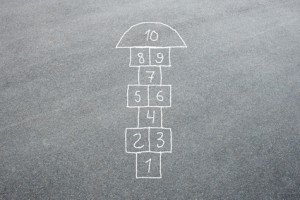Want to share your content on R-bloggers? click here if you have a blog, or here if you don't.Answers to the exercises are available here.

Exercise 1

if `x <- c(a = 1, b = 2,c=3,d=4)`
What is the output for the code:
`seq(5,11,along.with =x)`

Exercise 2

If `x= seq(4,12,4)` ,
what is the output for the code:
`rep(x,each=2)`

Exercise 3

What is the output for the code:
`seq(5,11,by=2,length.out=3)`

Exercise 4

What is the output for the code:
`rep(letters[1:10],3)`

Exercise 5

Create a sequence with values:
`100 95 90 85 80 75 70 65 60 55 50`

``` ```

Exercise 6

What is the output for the code:
`seq(10,0,by=5)`

Exercise 7

What is the output for the code:
`seq(2,10,by=4)==c(2,6,10)`

Exercise 8

What is the output for the code:
` rep(c('seq','rep'),each=4)`

Exercise 9

Consider two variables, A and B,
`A= as.Date("2016-11-01")`

`B = as.Date("2016-11-15")`

What is the output for the code:
`seq.Date(A,B, by = "1 day")`

Exercise 10
Consider two variables, C and D,

`C= as.Date("2016-02-01")`

`D = as.Date("2016-06-15")`

What is the output for the code:
`seq.Date(D,C, by = "-1 month")`

Want to practice regular sequences a bit more? We have more exercise sets on this topic here.